### IMO Shortlist 2005 problem C7

Kvaliteta:
Avg: 0.0
Težina:
Avg: 9.0
Suppose that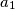$a_1$,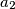$a_2$,$\ldots$,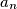$a_n$ are integers such that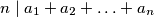$n\mid a_1 + a_2 + \ldots + a_n$.
Prove that there exist two permutations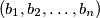$\left(b_1,b_2,\ldots,b_n\right)$ and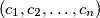$\left(c_1,c_2,\ldots,c_n\right)$ of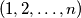$\left(1,2,\ldots,n\right)$ such that for each integer$i$ with$1\leq i\leq n$, we have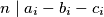Source: Međunarodna matematička olimpijada, shortlist 2005